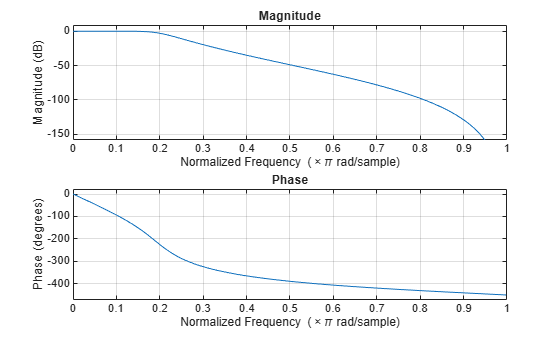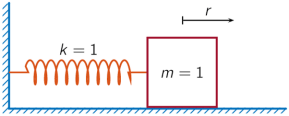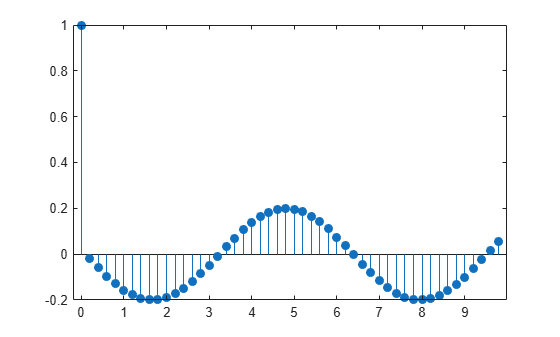# ss2sos

Convert digital filter state-space parameters to second-order sections form

## Syntax

``[sos,g] = ss2sos(A,B,C,D)``
``[sos,g] = ss2sos(A,B,C,D,iu)``
``[sos,g] = ss2sos(A,B,C,D,order)``
``[sos,g] = ss2sos(A,B,C,D,iu,order)``
``[sos,g] = ss2sos(A,B,C,D,iu,order,scale)``
``sos = ss2sos(___)``

## Description

example

````[sos,g] = ss2sos(A,B,C,D)` returns second-order section form `sos` with gain `g` that is equivalent to the state-space system represented by input arguments `A`, `B`, `C`, and `D`. The input state-space system must be single-output and real.```
````[sos,g] = ss2sos(A,B,C,D,iu)` specifies index `iu` that indicates which input of the state-space system `A`, `B`, `C`, `D` the function uses in the conversion.```
````[sos,g] = ss2sos(A,B,C,D,order)` specifies the order of the rows in `sos` with `order`.```
````[sos,g] = ss2sos(A,B,C,D,iu,order)` specifies both the index `ui` and the order of the rows `order`.```
````[sos,g] = ss2sos(A,B,C,D,iu,order,scale)` specifies the desired scaling of the gain and the numerator coefficients of all second-order sections.```
````sos = ss2sos(___)` embeds the overall system gain `g` in the first section. You can specify an input combination from any of the previous syntaxes.```

## Examples

collapse all

Design a fifth-order Butterworth lowpass filter, specifying a cutoff frequency of $0.2\pi$ rad/sample and expressing the output in state-space form. Convert the state-space result to second-order sections. Visualize the frequency response of the filter.

```[A,B,C,D] = butter(5,0.2); sos = ss2sos(A,B,C,D)```
```sos = 3×6 0.0013 0.0013 0 1.0000 -0.5095 0 1.0000 1.9996 0.9996 1.0000 -1.0966 0.3554 1.0000 2.0000 1.0000 1.0000 -1.3693 0.6926 ```
`freqz(sos)`A one-dimensional discrete-time oscillating system consists of a unit mass, $m$, attached to a wall by a spring of unit elastic constant. A sensor measures the acceleration, $a$, of the mass.The system is sampled at ${F}_{s}=5$ Hz. Generate 50 time samples. Define the sampling interval $\Delta t=1/{F}_{s}$.

```Fs = 5; dt = 1/Fs; N = 50; t = dt*(0:N-1);```

The oscillator can be described by the state-space equations

`$\begin{array}{c}x\left(k+1\right)=Ax\left(k\right)+Bu\left(k\right),\\ y\left(k\right)=Cx\left(k\right)+Du\left(k\right),\end{array}$`

where $x={\left(\begin{array}{cc}r& v\end{array}\right)}^{T}$ is the state vector, $r$ and $v$ are respectively the position and velocity of the mass, and the matrices

`$A=\left(\begin{array}{cc}\mathrm{cos}\Delta t& \mathrm{sin}\Delta t\\ -\mathrm{sin}\Delta t& \mathrm{cos}\Delta t\end{array}\right),\phantom{\rule{1em}{0ex}}B=\left(\begin{array}{c}1-\mathrm{cos}\Delta t\\ \mathrm{sin}\Delta t\end{array}\right),\phantom{\rule{1em}{0ex}}C=\left(\begin{array}{cc}-1& 0\end{array}\right),\phantom{\rule{1em}{0ex}}D=\left(\begin{array}{c}1\end{array}\right).$`

```A = [cos(dt) sin(dt);-sin(dt) cos(dt)]; B = [1-cos(dt);sin(dt)]; C = [-1 0]; D = 1;```

The system is excited with a unit impulse in the positive direction. Use the state-space model to compute the time evolution of the system starting from an all-zero initial state.

```u = [1 zeros(1,N-1)]; x = [0;0]; for k = 1:N y(k) = C*x + D*u(k); x = A*x + B*u(k); end```

Plot the acceleration of the mass as a function of time.

`stem(t,y,'filled')`Compute the time-dependent acceleration using the transfer function to filter the input. Express the transfer function as second-order sections. Plot the result.

```sos = ss2sos(A,B,C,D); yt = sosfilt(sos,u); stem(t,yt,'filled')```The result is the same in both cases.

## Input Arguments

collapse all

State matrix, specified as a matrix. If the system has p inputs and q outputs and is described by n state variables, then `A` is of size n-by-n.

Input-to-state matrix, specified as a matrix. If the system has p inputs and q outputs and is described by n state variables, then `B` is of size n-by-p.

Output-to-state matrix, specified as a matrix. If the system has p inputs and q outputs and is described by n state variables, then `C` is of size q-by-n.

Feedthrough matrix, specified as a matrix. If the system has p inputs and q outputs and is described by n state variables, then `D` is of size q-by-p.

Index, specified as an integer.

Row order in `sos`, specified as one of these values:

• `'down'` — Order the sections so that the first row of `sos` contains the poles that are closest to the unit circle.

• `'up'` — Order the sections so that the first row of `sos` contains the poles that are farthest from the unit circle.

The zeros are paired with the poles that are closest to them.

Scaling of the gain and numerator coefficients, specified as one of these values:

• `'none'` — Apply no scaling.

• `'inf'` — Apply infinity-norm scaling.

• `'two'` — Apply 2-norm scaling.

Using infinity-norm scaling in conjunction with `up`-ordering minimizes the probability of overflow in the realization. Using 2-norm scaling in conjunction with `down`-ordering minimizes the peak round-off noise.

Note

Infinity-norm and 2-norm scaling are appropriate for only direct-form II implementations.

## Output Arguments

collapse all

Second-order section representation, returned as a matrix. `sos` is an L-by-6 matrix of the form

`$\text{sos}=\left[\begin{array}{cccccc}{b}_{01}& {b}_{11}& {b}_{21}& 1& {a}_{11}& {a}_{21}\\ {b}_{02}& {b}_{12}& {b}_{22}& 1& {a}_{12}& {a}_{22}\\ ⋮& ⋮& ⋮& ⋮& ⋮& ⋮\\ {b}_{0L}& {b}_{1L}& {b}_{2L}& 1& {a}_{1L}& {a}_{2L}\end{array}\right]$`

whose rows contain the numerator and denominator coefficients bik and aik of the second-order sections of H(z), which is given by

`$H\left(z\right)=g\prod _{k=1}^{L}{H}_{k}\left(z\right)=g\prod _{k=1}^{L}\frac{{b}_{0k}+{b}_{1k}{z}^{-1}+{b}_{2k}{z}^{-2}}{1+{a}_{1k}{z}^{-1}+{a}_{2k}{z}^{-2}}$`

Overall system gain, returned as a real-valued scalar.

If you call the function with one output argument, the function embeds the gain in the first section, H1(z), so that

`$H\left(z\right)=\prod _{k=1}^{L}{H}_{k}\left(z\right)$`

Note

Embedding the gain in the first section when scaling a direct-form II structure is not recommended and can result in erratic scaling. To avoid embedding the gain, use the function with two outputs: `sos` and `g`.

## Algorithms

The `ss2sos` function uses this four-step algorithm to determine the second-order section representation for an input state-space system.

1. Find the poles and zeros of the system given by `A`, `B`, `C`, and `D`.

2. Use the function `zp2sos`, which first groups the zeros and poles into complex conjugate pairs using the `cplxpair` function. `zp2sos` then forms the second-order sections by matching the pole and zero pairs according to these rules:

1. Match the poles that are closest to the unit circle with the zeros that are closest to those poles.

2. Match the poles that are next closest to the unit circle with the zeros that are closest to those poles.

3. Continue this process until all of the poles and zeros are matched.

The `ss2sos` function groups real poles into sections with the real poles that are closest to them in absolute value. The same rule holds for real zeros.

3. Order the sections according to the proximity of the pole pairs to the unit circle. The `ss2sos` function normally orders the sections with poles that are closest to the unit circle last in the cascade. You can specify for `ss2sos` to order the sections in the reverse order by setting the order input to `'down'`.

4. Scale the sections by the norm specified by the `scale` input. For arbitrary H(ω), the scaling is defined by

`${‖H‖}_{p}={\left[\frac{1}{2\pi }\underset{0}{\overset{2\pi }{\int }}{|H\left(\omega \right)|}^{p}d\omega \right]}^{1/p}$`

where p can be either ∞ or 2. For details, see the references. This scaling is an attempt to minimize overflow or peak round-off noise in fixed-point filter implementations.

 Jackson, Leland B. Digital Filters and Signal Processing. Boston: Kluwer Academic Publishers, 1996.

 Mitra, Sanjit Kumar. Digital Signal Processing: A Computer-Based Approach. New York: McGraw-Hill, 1998.

 Vaidyanathan, P. P. “Robust Digital Filter Structures.” Handbook for Digital Signal Processing (S. K. Mitra and J. F. Kaiser, eds.). New York: John Wiley & Sons, 1993.